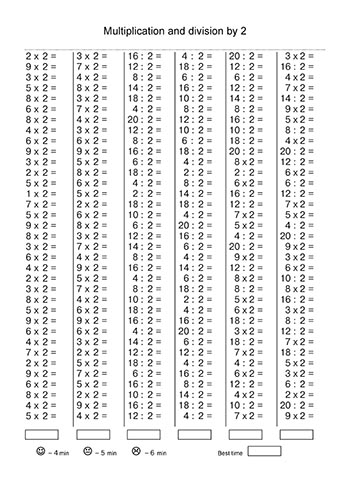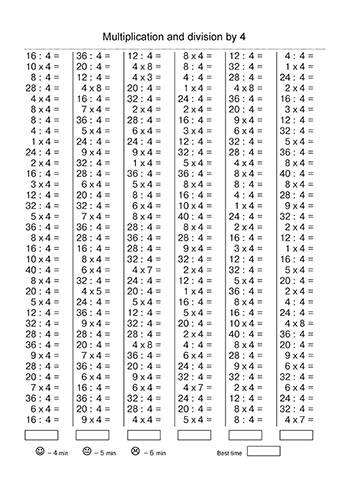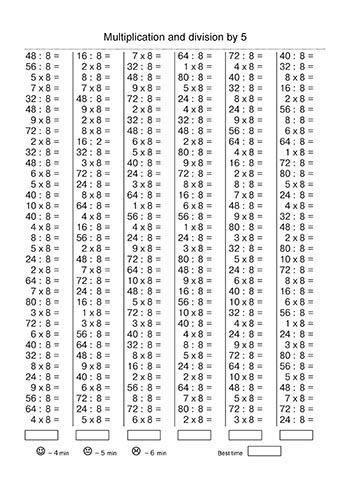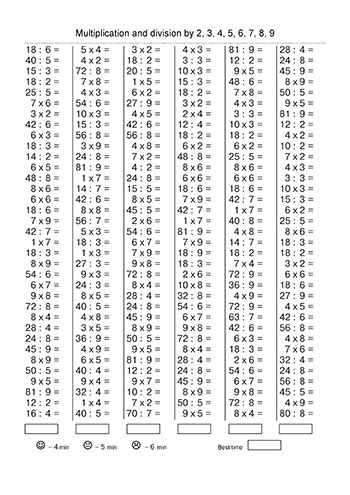0
(0)

To test and reinforce your child’s knowledge of multiplication and division of prime numbers, use the following templates, which contain many examples mixed up. The examples are ready for printing on A4 sheets and are divided into multiplication and division by certain numbers of the multiplication table.

Each card with examples contains a time that will determine the degree of preparation of the child in this section of mathematics.

#### Multiplication and division by 3#### Multiplication and division by 5#### Multiplication and division by 7#### Multiplication and division by 9#### Multiplication and division by 2, 3, 4, 5, 6, 7, 8, 9How useful was these post?

Click on a star to rate it!

Average rating 0 / 5. Vote count: 0

No votes so far! Be the first to rate these post.